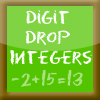Custom Search

# Find Our Cool Math Games to Play by Category:

### 7.NS.A.2a

Understand that multiplication is extended from fractions to rational numbers by requiring that operations continue to satisfy the properties of operations, particularly the distributive property, leading to products such as (–1)(–1) = 1 and the rules for multiplying signed numbers. Interpret products of rational numbers by describing real-world contexts.CCSS: 7.NS.A.1b, 7.NS.A.1c, 7.NS.A.1d, 7.NS.A.2a, 7.NS.A.2b, 7.NS.A.2c
Drag and drop the positive or negative integer that will complete the number sentence correctly Play using addition, subtraction, multiplication or division.CCSS: 7.NS.A.1b, 7.NS.A.1c, 7.NS.A.2a, 7.NS.A.2b
The integers version of Math Balloons.  Remove balloons to make groups of same colored balloons by determining if the answers to the problems using positive and negative integers are correct or incorrect.